Next: Projective Transformations Up: Important concepts from projective Previous: Homogeneous Coordinates

# Manipulating Points and Lines

This section describes some basic operations for manipulating points and lines in homogeneous coordinates.

(a) Computing a line through two points. Using Equation (5), the line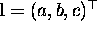which passes through points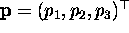and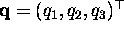must satisfy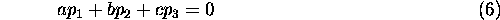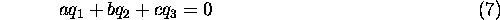The simplest way to remember the solution to these equations is as a cross-product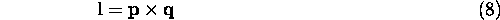(b) Computing the point of concurrency of two lines. Using Equation (5), the point of concurrencyof the lines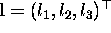and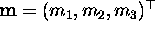must satisfy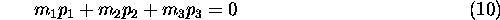Note how the formulation of this problem mirrors problem (a). This is a manifestation of the principle of duality - in any construction or theorem involving points and lines on the projective plane, each instance of a point can be exchanged for an instance of a line, and each instance of a line exchanged for an instance of a point, and the result is a dual construction or theorem to the original one (in particular this is useful because for any theorem which is true, its dual is also true).Thus, this is the dual problem to (b) and is solved in the same way,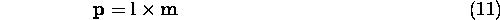Remaining operations in this section are not part of projective geometry. They have been included because they are of practical use.

(c) Normalising homogeneous coordinates. The manipulations of points and lines may result in homogeneous coordinates with very large or very small magnitudes. This can cause numerical error so it is a good idea to normalise homogeneous coordinates at each stage of a computation. Normalisation can be carried out in a straightforward way since a homogeneous vector can be multiplied by any scalar and it will still represent the same point or line. A suggested normalisation for a point is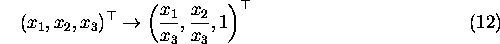and for a lineThe denominator can be zero in both Equations (12) and (13), in which case any alternative normalisation which is convenient for the associated application can be used instead. The reason for the particular form of the line normalisation is to produce simple formulae in the operations inand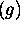below.

(d) Computing a line through many points. Computing a line through two points as described in (a) involves the solution of two equations in two unknowns (although the equations actually contain three unknowns a, b, c, it is only the ratiosand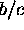which are significant because the scale of the homogeneous vector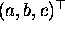is arbitrary). Given n points, there are n equations in 2 unknowns and the system can be solved using a least-squares method.

Two approaches were investigated for this thesis - orthogonal regression and Kanatani's ``moment matrix'' [Kanatani 1992]. In the latter, the computation of different weightings for individual points in the way described by Kanatani was not implemented. These methods produced slightly different results but neither one was consistently better than the other.

(e) Computing the point of concurrency of many lines. This is the dual problem to (d), and is solved in the same way.

(f) Computing the perpendicular distance from a line to a point. Normalise the line and point as described in (c). If the line vector isand the point vector is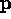, the perpendicular distance is given by the dot product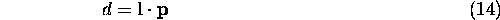And, when the line and point are incident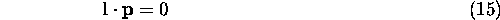Equation (14) has a simple form as a result of the particular normalisation applied toand- see [Faux 1979] for the equation for the general case.

(g) Computing the angle between two lines. Normalise the lines as described in (c). If the normalised lines are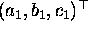and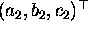, the angle between them is given byEquation (16) has a simple form as a result of the particular normalisation applied to the two lines - see [Faux 1979] for the equation for the general case.Next: Projective Transformations Up: Important concepts from projective Previous: Homogeneous Coordinates

Bob Fisher
Fri Nov 7 12:08:26 GMT 1997